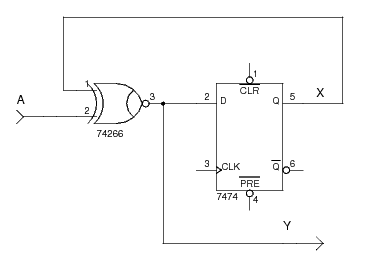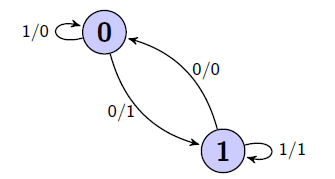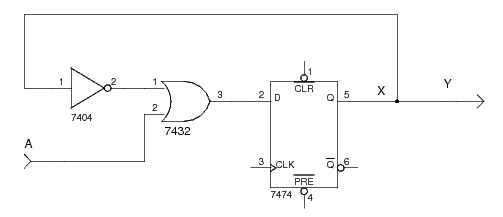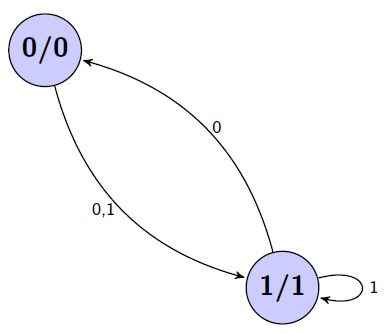# State Diagram

A state diagram shows the current state, inputs, next state, and outputs of a sequential logic circuit in a graphical form. It shows the same information as can be found in a state table.

## Example 1

Consider the following Mealy model sequential logic circuit containing a single D flip-flop (not all connections are shown to keep the schematic simple):The sole input is `A` and the sole output is `Y`. With one flip-flop we have two (21=2) different states (`X=0` and `X=1`). We can write the flip-flop input equation for the D flip-flop with output `X` as: `DX = A xnor X`. The Boolean equation for the output is `Y = A xnor X`. Using these we can create the following table:

Current
State
InputNext
State
Output
`X` `A` `X` `Y`
`0` `0` `1` `1`
`0` `1` `0` `0`
`1` `0` `0` `0`
`1` `1` `1` `1`

The equivalent state diagram is as follows:The nodes are marked with the circuit states and the edges are marked `A/B` which mean "input `A`" and "output `B`". So, in state `0`, if we have input `0` then we generate output `1` and move to state `1`. This coincides with the first row of the state table.

## Example 2

Consider the following Moore model sequential logic circuit containing a single D flip-flop (not all connections are shown to keep the schematic simple):The sole input is `A` and the sole output is `Y`. With one flip-flop we have two (21=2) different states (`X=0` and `X=1`). We can write the flip-flop input equation for the D flip-flop with output `X` as: `DX = A or not(X)`. The Boolean equation for the output is `Y = X`. Using these we can create the following table:

Current
State
InputNext
State
Output
`X` `A` `X` `Y`
`0` `0` `1` `0`
`0` `1` `1` `0`
`1` `0` `0` `1`
`1` `1` `1` `1`

The equivalent state diagram is as follows:The nodes are marked with the circuit states and their outputs. The edges between states are marked with the inputs. So, in state `0`, we always generate output `0` and if we have input `0` or `1`, then we move to state `1`. This coincides with the first two rows of the state table.

## References

Mano, M. Morris, and Kime, Charles R. Logic and Computer Design Fundamentals. 2nd Edition. Prentice Hall, 2000.